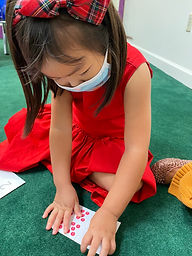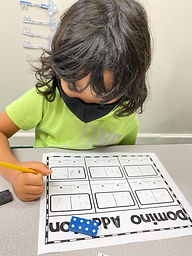## Ms. Brianne

### Target 1​

###### Lesson Type:

New

Number Operation

:

Number Meaning

Understand the relationship between numbers and quantities.

###### 1:

Use one-to-one correspondence when counting to connect one quantity with one number name.

###### 2:

Understand that each successive number in the counting sequence is one larger than the previous number.

###### 3:

Count objects to determine how many there are.

Kindergarden

###### Vocabulary:

Number, Count

Activities:

• Students flipped over a card and covered the matching number on a number grid.
• Students used one-to-one correspondence as they counted dots on a ten-frame and named how many there were.
• Students put together number puzzles by matching numerals with pictures of the corresponding quantities.### Home Exploration

###### Guiding Questions:## Absent Students:

### Target 2

:

###### 1:

Understand that combining numbers creates a larger quantity.

###### 2:

Represent “putting together” with objects, fingers, drawings, etc.

Kindergarden

###### Vocabulary:

Activities:

Students used dominoes to generate the two parts in an addition equation. They wrote the matching equation and counted to find the total.### Home Exploration

###### Guiding Questions:### Target 3

:

###### 1:

Create a pattern based on a given rule (ABAB, AABB, AAB, ABB, ABC, ABCD).

Kindergarden

###### Vocabulary:

Pattern, Repeat

Activities:

• Students used cuisinaire rods to create different patterns (AB, AAB, ABB, ABC, ABCD).
• Students created pattern headbands with a shape or color pattern.### Home Exploration